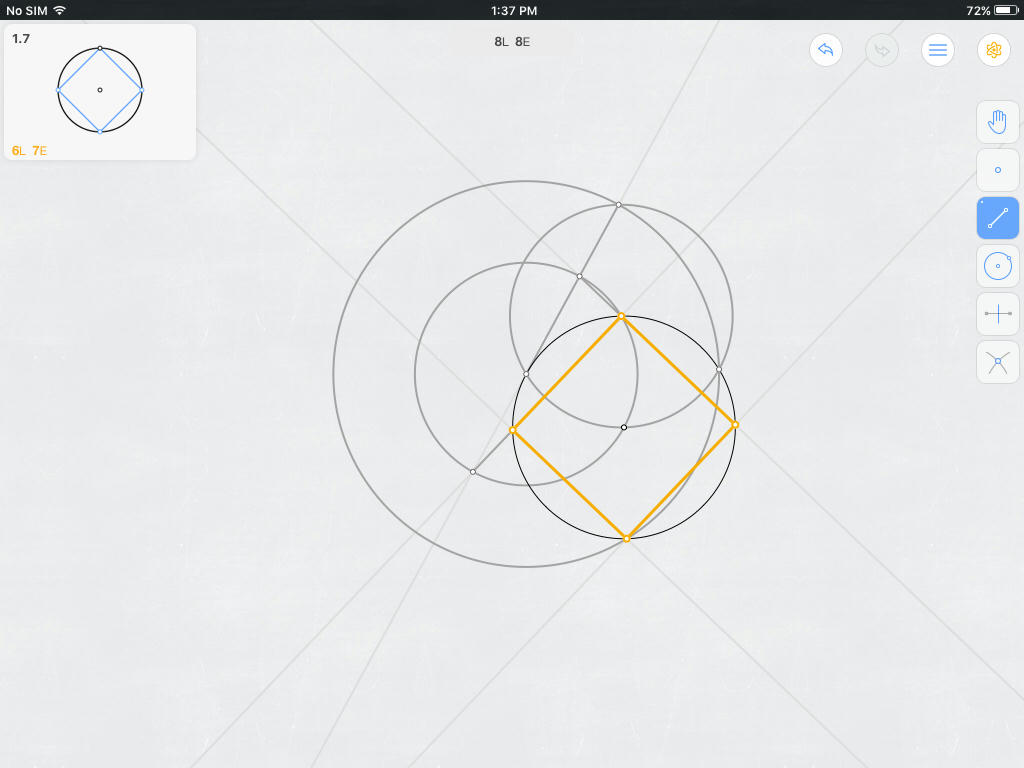# Inscribing square in circle in just seven compass-and-straightedge steps

Problem Here is one of the challenges posed on Euclidea, a mobile app for Euclidean constructions: Given a $\circ O$ centered on point $O$ with a point $A$ on it, inscribe $\square{ABCD}$ within the circle — in just seven elementary steps. Euclidea hints that the first two steps use the compass, the third uses the straightedge, and the last four use the straightedge to draw the sides themselves of $\square{ABCD}$.

Definitions The problem is not considered solved until each line containing one of the four sides of the desired square are drawn; merely finding the vertices of the square does not suffice. Naming and creating points are of course allowed and fortunately do not count toward the seven elementary steps allowed in this problem. Other than these ‘zero-step’ steps, Euclidea permits only two elementary steps, each costing one step:

1. Create an infinite line connecting two points using an unmarked, one-dimensional, infinitely long straightedge. (Even merely extending a given line segment costs one step.)
2. Create a circle using a compass that collapses immediately thereafter.

Research of previous Mathematics Stackexchange questions I am aware that there is a seven-step process previously described at How can I construct a square using a compass and straight edge in only 8 moves?. Notwithstanding the post’s title, it actually has just seven steps since its first corresponds to constructing the given $\circ O$. This solution fails, however, since the resulting inscribed square is neither inscribed in the given $\circ O$ nor inclusive of the given point $A$ As a vertex.

Attempt 1: 8-step solution using perpendicular bisectors

1. Take one step to extend $\overline{AO}$ to the other side of $\circ O$.
• Take as point $C$ the new intersection between said line and circle.
2. Take three steps to define $L$, the perpendicular bisector of diameter $\overline{AC}$.
• Take as points $B$ and $D$ the intersections of $L$ with $\circ O$.
3. Take four steps to draw the sides themselves of $\square{ABCD}$.

Attempt 2: 8-step solution using a 15-75-90 triangle It turns out that @Blue’s successful 7-step solution uses much the same circles and 15-75-90 triangle as the one proposed here.1. Take one step to create $\circ A$ with radius $AO$.
• Take as point $E_1$ the ‘left’ resulting point of intersection.
• Take as point $E_2$ the ‘right’ resulting point of intersection.
2. Take one step to create $\circ P$ with radius $E_1O$.
3. Take one step to create $\circ Q$ with radius $E_1E_2$.
• Take as point $C$ the intersection point between circle $Q$ and circle $O$.
• Take as point $F$ the intersection point between circle $Q$ and circle $A$.
4. Take one step to create $\overleftrightarrow{E_1F}$.
• Take as point $G_1$ the resulting ‘top’ intersection point with $\circ P$.
• Take as point $G_2$ the resulting ‘bottom’ intersection point with $\circ P$.
5. Take one step to create $\overleftrightarrow {AG_1}$ to effectively draw $\overline{AB}$.
• Take as point $B$ the resulting intersection between said line and $\circ O$.
6. Take one step to create $\overline{BC}$.
7. Take one step to create $\overleftrightarrow{AG_2}$ to effectively draw $\overline{AD}$.
• Take as point $D$ the resulting intersection between said line and $\circ O$.
8. Take one step to create $\overline{CD}$.
• This completes desired $\square ABCD$, albeit in one too many steps.1. Construct $$◯A\bigcirc A$$ through $$OO$$.
• Let $$P1P_1$$ and $$P2P_2$$ be the points where $$◯A\bigcirc A$$ meets $$◯O\bigcirc O$$.
1. Construct $$◯P1\bigcirc P_1$$ through $$P2P_2$$.
• Let $$CC$$ be the (other) point where $$◯P1\bigcirc P_1$$ meets $$◯O\bigcirc O$$.
1. Construct $$↔OP1\overleftrightarrow{OP_1}$$.
• Let $$Q1Q_1$$ and $$Q2Q_2$$ be the points where $$↔OP1\overleftrightarrow{OP_1}$$ meets $$◯P1\bigcirc P_1$$.
1. Construct $$↔CQ1\overleftrightarrow{CQ_1}$$.
• Let $$BB$$ be the point where $$↔CQ1\overleftrightarrow{CQ_1}$$ meets $$◯O\bigcirc O$$.
• Note that we have constructed $$↔BC\overleftrightarrow{BC}$$.
1. Construct $$↔CQ2\overleftrightarrow{CQ_2}$$.
• Let $$DD$$ be the point where $$↔CQ2\overleftrightarrow{CQ_2}$$ meets $$◯O\bigcirc O$$.
• Note that we have constructed $$↔CD\overleftrightarrow{CD}$$.
1. Construct $$↔AB\overleftrightarrow{AB}$$.
2. Construct $$↔AD\overleftrightarrow{AD}$$.

Square $$◻ABCD\square ABCD$$, with constructed edge-lines, is inscribed in $$◯O\bigcirc O$$. (Proof that the quadrilateral is, in fact, a square, is left as an exercise to the reader.)

Edit. Having been asked to elaborate on the square

• As of Step 2, we know $$△P1P2C\triangle P_1 P_2 C$$ is equilateral and that $$¯OA\overline{OA}$$ is on the perpendicualr bisector of side $$¯P1P2\overline{P_1 P_2}$$. Therefore, $$¯AC\overline{AC}$$ is a diameter of $$◯O\bigcirc O$$, and we have that $$∠ABC\angle ABC$$ and $$∠ADC\angle ADC$$ (for point $$DD$$ constructed later) are right angles by Thales’ Theorem.

• As of Step 4, as observed by Jan and Tristan in the comments, $$¯Q1Q2\overline{Q_1 Q_2}$$ is a diameter of $$◯P1\bigcirc{P_1}$$, so $$∠Q1CQ2\angle Q_1 C Q_2$$ is a right angle. Therefore, $$◻ABCD\square ABCD$$ is at least a rectangle.

• Now, define $$a:=|¯OA|a := |\overline{OA}|$$, so that $$|¯P1P2|=a√3|\overline{P_1P_2}| = a\sqrt{3}$$ and $$|¯OQ1|=a(1+√3)|\overline{OQ_1}| = a( 1 + \sqrt{3})$$. Since $$∠AOQ1=60∘\angle AOQ_1 = 60^\circ$$, if we let $$RR$$ be the foot of the perpendicular from $$Q1Q_1$$ to $$↔OA\overleftrightarrow{OA}$$, then $$|¯OR|=a2(1+√3)|\overline{OR}| = \frac{a}{2}( 1 + \sqrt{3})$$ and
$$|¯Q1R|=a√32(1+√3)=a2(3+√3)=a+|¯OR|=|¯CR||\overline{Q_1R}| = \frac{a \sqrt{3}}{2}(1+\sqrt{3}) = \frac{a}{2}(3 + \sqrt{3}) = a + |\overline{OR}| = |\overline{CR}|$$ Thus, $$∠Q1CR=45∘\angle Q_1 C R = 45^\circ$$ and we may conclude that $$◻ABCD\square ABCD$$ is a square. $$◻\square$$